1. One of the following numbers is prime. Which is it?

A:B:C:D:E:2. Last year, an earthworm from Wigan named Dave wriggled into the record books as the largest found in the UK. Dave was 40 cm long and had a mass of 26 g.

What was Dave’s mass per unit length?

A:B:C:D:E:3. The five integers 2, 5, 6, 9, 14 are arranged into a different order. In the new arrangement, the sum of the first three integers is equal to the sum of the last three integers.

What is the middle number in the new arrangement?

A:B:C:D:E:4. Which of the following is equal to $2017$ - $\dfrac{1}{2017} %speech% 1 divided by 2017.$

A:B:C:D:E: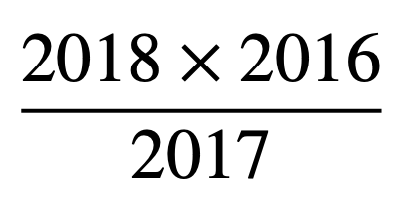5. One light-year is nearly $6\times10^{12}$ miles. In $2016$, the Hubble Space Telescope set a new cosmic record, observing a galaxy $13.4$ thousand million light-years away.

Roughly how many miles is that?

A:B:C:D:E:6. The circles in the diagram are to be coloured so that any two circles connected by a line segment have different colours.

What is the smallest number of colours required?

A:B:C:D:E:7. The positive integer $k$ satisfies the equation $\sqrt{2}0+\sqrt{8}+\sqrt{18}$ = $\sqrt{k}$.

What is the value of $k$ ?

A:B:C:D:E:8.  When evaluated, which of the following is not an integer?

A: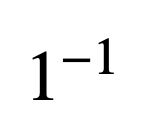B: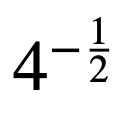C: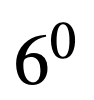D: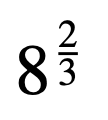E: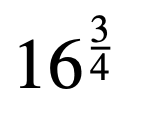9. The diagram shows an n× (n+1) rectangle tiled with k× (k+1) rectangles, where n and k are integers and k takes each value

from 1 to 8 inclusive.

What is the value of n?

A:B:C:D:E:10. A rectangle is divided into three smaller congruent rectangles as shown.

Each smaller rectangle is similar to the large rectangle.

In each of the four rectangles, what is the ratio of the length of a longer side to that of a shorter side?

A: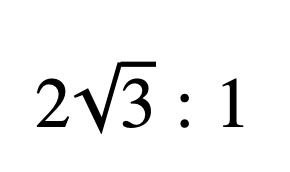B: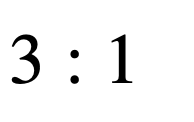C: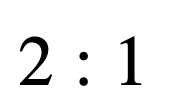D: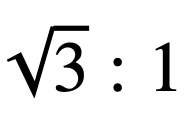E: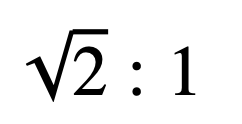11. The teenagers Sam and Jo notice the following facts about their ages:

The difference between the squares of their ages is four times the sum of their ages. The sum of their ages is eight times the difference between their ages.

What is the age of the older of the two?

A:B:C:D:E:12. The diagram shows a square and a regular decagon that share an edge. One side of the square is extended to meet an extended edge of the decagon.

What is the value of x?

A:B:C:D:E:A:B:C:D:E:A:B:C:D:E:1. The diagram shows a square PQRS. Points T, U, V and W lie on the edges of the square as shown, such that PT = 1, QU = 2, RV = 3 and SW = 4

The area of TUVW is half that of PQRS. What is the length of PQ?

A:B:C:D:E:Back to the top